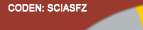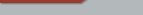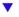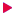| Home  | About ScienceAsia  | Publication charge  | Advertise with us  | Subscription for printed version  | Contact usEditorial Board Journal Policy Instructions for Authors Online submission Author Login Reviewer Login Volume 47 Number 6 Volume 47 Number 5 Volume 47 Number 4 Volume 47S Number 1 Volume 47 Number 3 Volume 47 Number 2 Earlier issuesVolume 44 Number 3Volume 44 Number 4 Volume 44 Number 5previous article next articleResearch articles

ScienceAsia 44 (2018): 288-291 |doi: 10.2306/scienceasia1513-1874.2018.44.288

# On a generalization of transformation semigroups that preserve equivalences

## Nares Sawatraksa*, Chaiwat Namnak

ABSTRACT:     Let T(X) be the full transformation semigroup on a nonempty set X. For an equivalence relation σ on X, Pei introduced and studied the subsemigroup of T(X) defined by T(X,σ) = {α ∈ T(X) : ∀x, y ∈ X,(x, y) ∈ σ implies (xα, yα) ∈ σ}, which is called a transformation semigroup preserving the equivalence σ. In this paper, for two equivalence relations σ, ρ with ρ ⊆ σ on a nonempty set X, we introduce the subsemigroup T(X,σ,ρ) = {α ∈ T(X) : ∀x, y ∈ X,(x, y) ∈ σ implies (xα, yα) ∈ ρg of T(X) which generalizes the notation of the subsemigroup T(X,σ) of T(X). A necessary and sufficient condition under which T(X,σ,ρ) is a BQ-semigroup (a semigroup whose biideals and quasi-ideals coincide) is given. We also prove that T(X,σ) of T(X) can be embedded into a semigroup of T(Y, Z) = {α ∈ T(Y ) : Y α ⊆ Z} for some sets Y and Z with Z ⊆ Y .